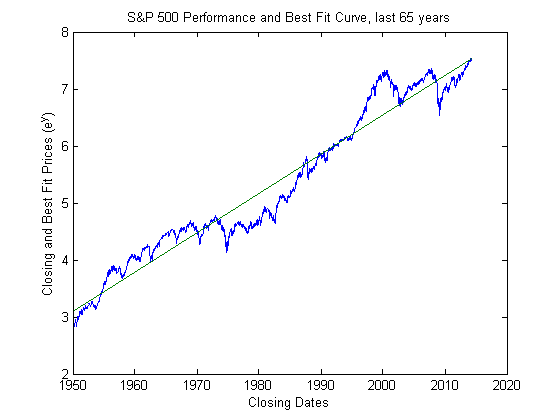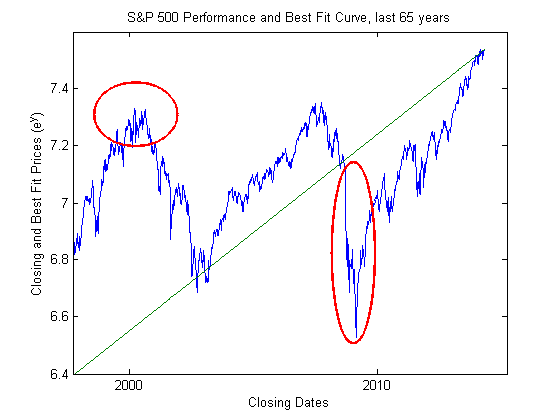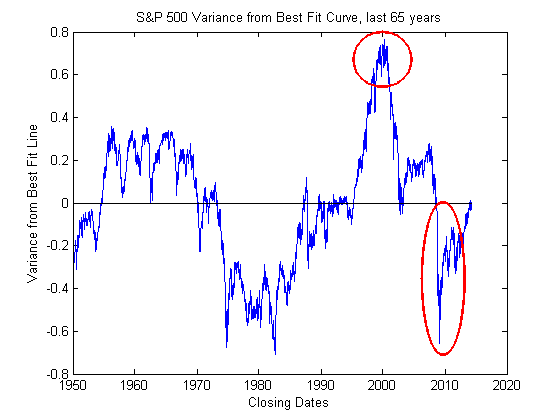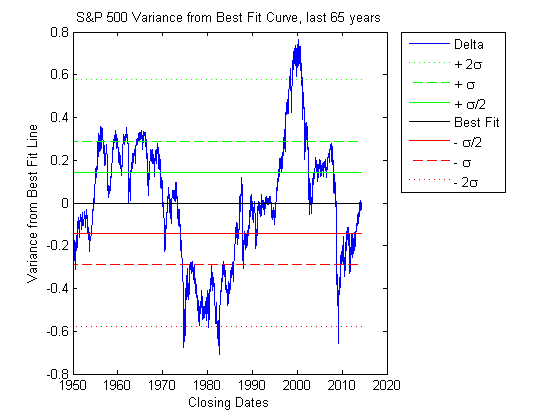## Loren on the Art of MATLABTurn ideas into MATLAB

Note

Loren on the Art of MATLAB has been archived and will not be updated.

# Long-term Analysis of the S&P 500

Here at the MathWorks we have a great staff to support the financial industry. We also have our fair share of DIYers who like to follow the market and manage their own investments, such as this edition's guest blogger, Steve Cruickshank, an admitted "cocktail party economist".

During his day job, Steve is the marketing manager for our MATLAB Application Deployment products.

### Contents

#### Introduction - Long term tracking and statistical analysis of the S&P 500

If you’re an amateur investor like me, you probably experienced great frustration during late 2008 and early 2009, watching the value of your various stock portfolios, IRAs, and 401K accounts plummet. Sure, we lived the high life during the run-ups of the late 90s dot-com boom and subsequent gains during 2003 – 2008, but the Great Recession of late 2008 and early 2009 made me take a closer look at the S&P 500, especially the long term trends.

Caveat emptor - I’m not a financial industry professional and couldn’t tell you the difference between GARCH and Black-Scholes without using Wikipedia, but I do enjoy managing my own investments and crunching numbers to gain perspective. As long as we’re in “full disclosure” mode, I borrowed the phrase “cocktail party economist” from my grad school Economics professor.

When looking at the S&P 500 trends over various timeframes, or reading web pages from the various pundits, it’s easy to see that you can cherry pick a time period to validate virtually any hypothesis. Think the market is below a reasonable level? Then start your analysis fifteen years ago … 1999 … the height of the dot com boom. Since then the S&P has grown a paltry 1.4% annually, far below the historical average annual growth rate of ~7.5%. Plenty of room for growth, so buy everything in sight.

Want to espouse the theory that the market has grown way too fast and is in yet another bubble just waiting to burst? Sure, we can do that! Start your analysis five years ago … early 2009 … the bottom of the financial crisis. Since then the S&P has grown at a ridiculous rate, over 20% annually, roughly the same as during the dot com boom years. Time to sell everything.

Given those obvious extremes, perhaps the best way to eliminate the false conclusions reached by cherry picking time periods is to analyze data from as far back as possible and evaluate the current market based on the long term trends. The example uses Yahoo Finance to provide data for the S&P 500 since 1950, but you could use other data sources and time frames as you see fit.

The MathWorks' software used in this example is MATLAB and the Datafeed Toolbox. Home users may want to use MATLAB Home and hard-core number crunchers may prefer the advanced functionality provided by the Curve Fitting Toolbox.

#### Setting the initial constraints

I am using fetch from the Datafeed Toolbox to pull data from Yahoo based on a user specified timeframe, i.e. how many years from now. This example uses the 'w' option to get weekly data, but could also use 'm' for month or 'd' for day without materially affecting the results and conclusions.

% Set Yahoo as data source.
y = yahoo;

% Determine how far back to look. Yahoo provides data starting at 1950,
% so 65 years gathers all the available data.
years = 65;
start = now - (365.25 * years);

% Get data from Yahoo.
CloseData = fetch(y, '^GSPC', 'Close', start, now, 'w');


#### Curve fit calculations

We can then extract the closing price and date for each point, using the polyfit and polyval functions to determine the best fit line through the data.

% Extract data of interest from Yahoo results
ClosePrice = CloseData(:,2);
CloseDate = CloseData(:,1);

% Curve fit using a polynomial fit to create curve fit item and trend data
% based on curve fit result.
TrendLine = polyfit(CloseDate,log(ClosePrice), 1);
TrendData = polyval(TrendLine,CloseDate);


Option: If your analysis requires other types of curve fitting, you could also use Curve Fitting Toolbox, as shown here:

  TrendLine2 = fit(CloseDate, log(ClosePrice), 'poly1');
TrendData = feval(TrendLine2, CloseDate);

#### Plotting and visualizing

Plotting the variables CloseDate, ClosePrice, and TrendData on a log plot provides the following image. The variables plotted along the y-axis (ClosePrice and TrendData) are expressed as exponential functions, such as $ClosePrice = e^y$. Plotting data using exponents and/or log plots provides a better visual representation of average growth rates over long periods of time.

% Plotting the actual performance against best fit line
plot(CloseDate,log(ClosePrice),CloseDate,TrendData);
title(['S&P 500 Performance and Best Fit Curve, last ',num2str(years),' years'])
ylabel('Closing and Best Fit Prices (e^y)')
xlabel('Closing Dates')
datetick('x');This plot provides an effective visual mechanism to tell if the market is currently above or below the long term average. Interestingly, the current market (as of late-April 2014) is landing very close to the best fit curve, so perhaps the market is just about where it “should” be. You probably won’t hear that sentiment from market prognosticators because bulls, bears, and bubbles generate more page views.

Using the axis function to zoom in on the previous plot, we can highlight two easily recognizable data points: the peak of the late ‘90’s dot com bubble and the financial crisis roughly ten years later. In this example, the annotation function provides the graphics.

% Setting appropriate zoom coordinates
axis([729661, 736053, 6.4, 7.6])

% Create ellipses to highlight areas of interest
annotation('ellipse',[0.168, 0.652, 0.149, 0.152], 'LineWidth',2, 'Color','r');
annotation('ellipse',[0.592, 0.183, 0.078, 0.431], 'LineWidth',2, 'Color','r');#### Quantifying the data with statistics

Visuals are nice, but what if you, like so many of us here at MathWorks, prefer numbers and want to quantify the information rather than eyeball it? The first step in quantifying this data is to determine the variance, the variable Delta in my example, between the actual closing price and the projected price based on the best fit line.

% Calculate the difference between actual results and best fit curve
Delta = log(ClosePrice) - TrendData;


The calculations above will transpose the sloped line of the best fit curve to a horizontal line, but the familiar data points from the previous figure are clear, the dot com boom and financial crisis. We can then plot Delta relative to the best fit curve using the functions below. The zeros function converts a single '0' to a vector for effective plotting.

% Clear the figure then plot the raw data. Note that the BestFitCurve is
% multiplied by zeros to get reasonable legend
clf
h1 = plot(CloseDate, Delta);
title(['S&P 500 Variance from Best Fit Curve, last ',num2str(years),' years'])
datetick ('x');
BestFitCurve = line(CloseDate, zeros(size(CloseDate)), 'Color', 'k');
ylabel('Variance from Best Fit Line')
xlabel('Closing Dates')

% Create ellipses to highlight areas of interest
annotation('ellipse', [0.753, 0.155, 0.077, 0.364], 'LineWidth',2, 'Color','r');
annotation('ellipse', [0.631, 0.793, 0.104, 0.131], 'LineWidth',2, 'Color','r');To continue quantifying the results, we can use the std function to determine the standard deviation, variable name Sigma, for the variable Delta. To cover a broader range of variances we can then apply multipliers (2, 1, ½, -½, -1, -2) to Sigma, showing statistically how far above or below the market is with respect to the long term average. Thankfully none of the market fluctuations reached the Six Sigma levels.

% Define Sigma as the standard deviation of Delta, then convert to a vector
% using a matrix of ones
Sigma = std(Delta)*ones(size(CloseDate));

% Clear the previous figure, define the various Sigma's, then plot the data
% with a legend
clf
h1 = plot (CloseDate, Delta);
title(['S&P 500 Variance from Best Fit Curve, last ',num2str(years),' years'])
datetick('x');
BestFitCurve = line(CloseDate, zeros(size(CloseDate)), 'Color', 'k');
TwoSigma = line(CloseDate, 2*Sigma, 'Color', 'g', 'LineStyle', ':');
OneSigma = line(CloseDate, Sigma, 'Color', 'g', 'LineStyle', '--');
HalfSigma = line(CloseDate, 0.5*Sigma, 'Color', 'g');
NegHalfSigma = line(CloseDate, -0.5*Sigma, 'Color', 'r');
NegOneSigma = line(CloseDate, -Sigma, 'Color', 'r', 'LineStyle', '--');
NegTwoSigma = line(CloseDate, -2*Sigma, 'Color', 'r', 'LineStyle', ':');
ylabel('Variance from Best Fit Line')
xlabel('Closing Dates')

legend([h1 TwoSigma OneSigma HalfSigma BestFitCurve ...
NegHalfSigma NegOneSigma NegTwoSigma], ...
{'Delta', '+ 2\sigma', '+ \sigma', '+ \sigma/2' 'Best Fit', ...
'- \sigma/2', '- \sigma','- 2\sigma'}, 'Location', 'NorthEastOutside')#### Conclusions

With data and analysis like above, hindsight is always 20/20. In a perfect world, most investors like myself probably wish they had sold everything when the S&P went above the +2Sigma level near the end of the dot com bubble, and bought as much as possible on margin during extremely short window below -2Sigma in early 2009.

We all have our own risk profiles, so I won’t pretend to tell you what level represents an attractive buying or selling opportunity ... you need to do your own homework and decide for yourself. The example in this article is just one method to cut through the propaganda and utilize math to provide relatively unbiased data.

One final question for the readers ... do you agree with the statement made earlier that the current level of S&P 500 is about where it "should" be given the long term data? If not, what's your expected level and rationale? Please let me know here.

Published with MATLAB® R2014a